Скачать презентацию 1 of 54 © Boardworks Ltd 2007 AMOUNT

lesson_11-12_presentation.ppt

• Размер: 2 Mегабайта
• Количество слайдов: 25

## Описание презентации 1 of 54 © Boardworks Ltd 2007 AMOUNT по слайдам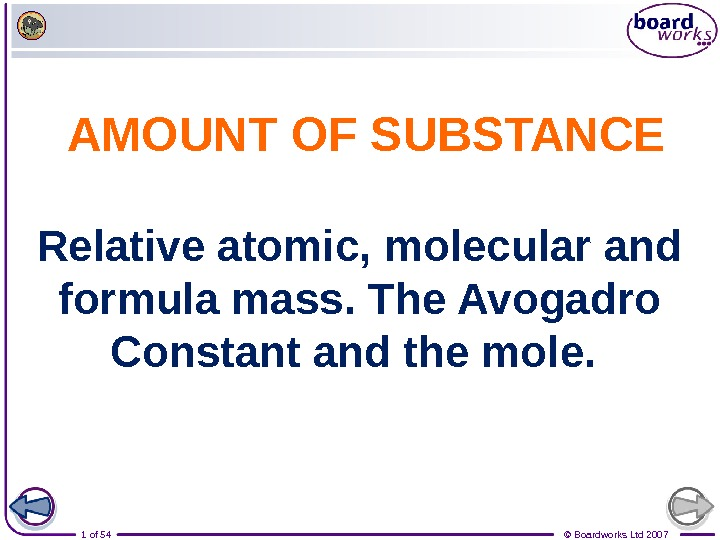1 of 54 © Boardworks Ltd 2007 AMOUNT OF SUBSTANCE Relative atomic, molecular and formula mass. The Avogadro Constant and the mole.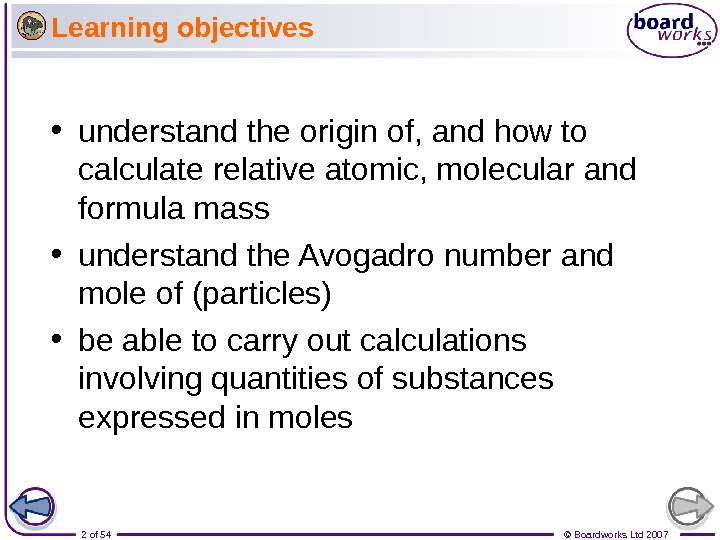2 of 54 © Boardworks Ltd 2007 Learning objectives • understand the origin of, and how to calculate relative atomic, molecular and formula mass • understand the Avogadro number and mole of (particles) • be able to carry out calculations involving quantities of substances expressed in moles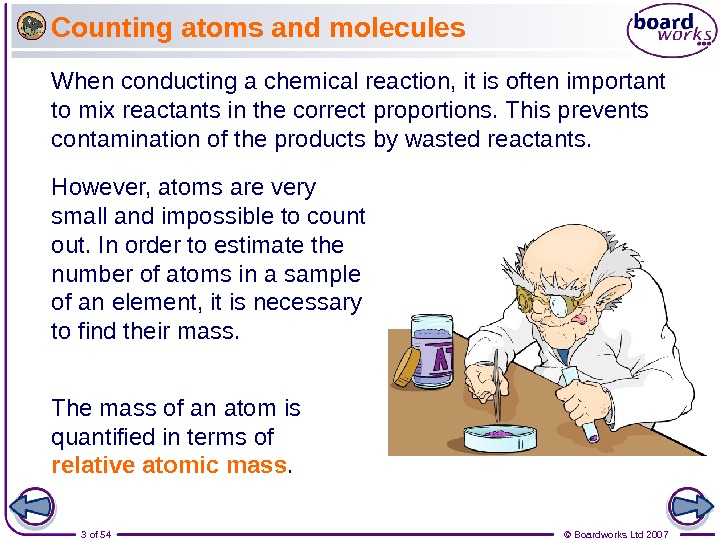3 of 54 © Boardworks Ltd 2007 Counting atoms and molecules When conducting a chemical reaction, it is often important to mix reactants in the correct proportions. This prevents contamination of the products by wasted reactants. However, atoms are very small and impossible to count out. In order to estimate the number of atoms in a sample of an element, it is necessary to find their mass. The mass of an atom is quantified in terms of relative atomic mass.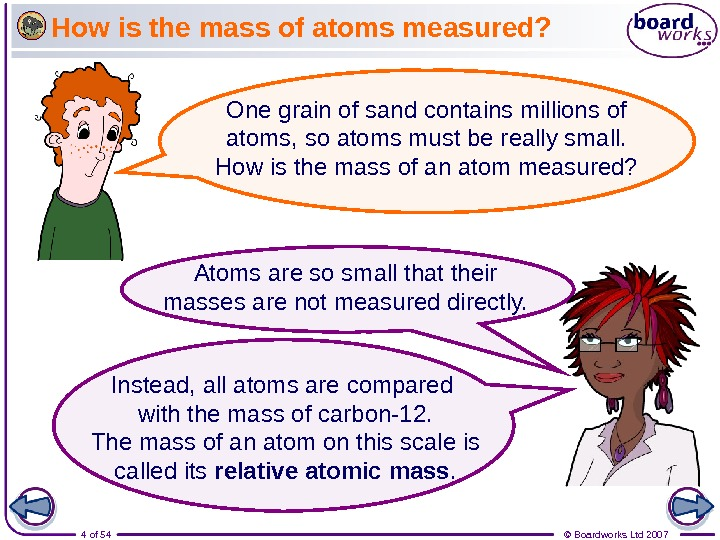4 of 54 © Boardworks Ltd 2007 How is the mass of atoms measured? Atoms are so small that their masses are not measured directly. One grain of sand contains millions of atoms, so atoms must be really small. How is the mass of an atom measured? Instead, all atoms are compared with the mass of carbon-12. The mass of an atom on this scale is called its relative atomic mass.5 of 54 © Boardworks Ltd 2007 What is relative atomic mass?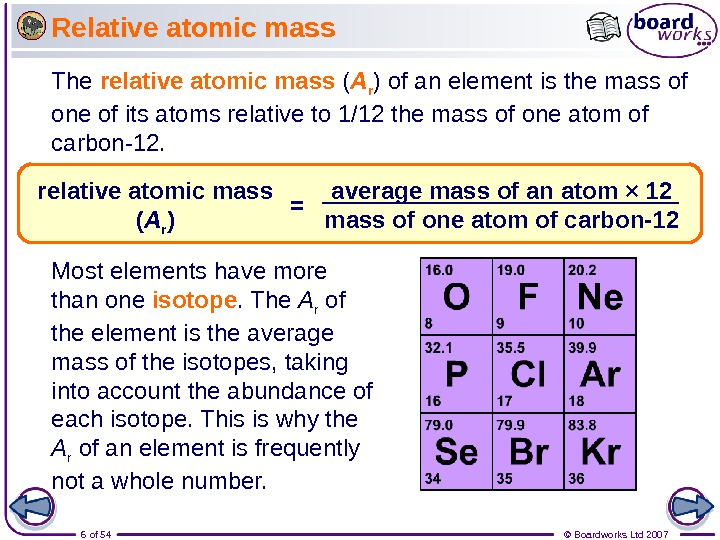6 of 54 © Boardworks Ltd 2007 Relative atomic mass The relative atomic mass ( A r ) of an element is the mass of one of its atoms relative to 1/12 the mass of one atom of carbon-12. Most elements have more than one isotope. The A r of the element is the average mass of the isotopes, taking into account the abundance of each isotope. This is why the A r of an element is frequently not a whole number. average mass of an atom × 12 mass of one atom of carbon-12 relative atomic mass ( A r ) =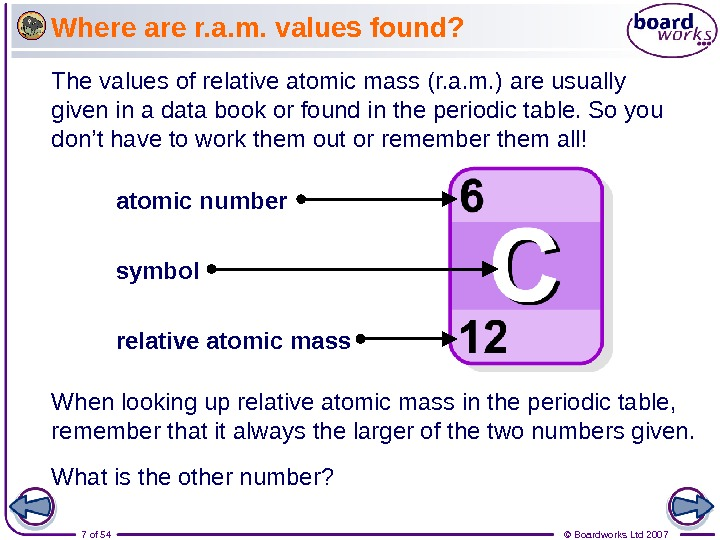7 of 54 © Boardworks Ltd 2007 Where are r. a. m. values found? The values of relative atomic mass (r. a. m. ) are usually given in a data book or found in the periodic table. So you don’t have to work them out or remember them all! When looking up relative atomic mass in the periodic table, remember that it always the larger of the two numbers given. atomic number symbol relative atomic mass What is the other number?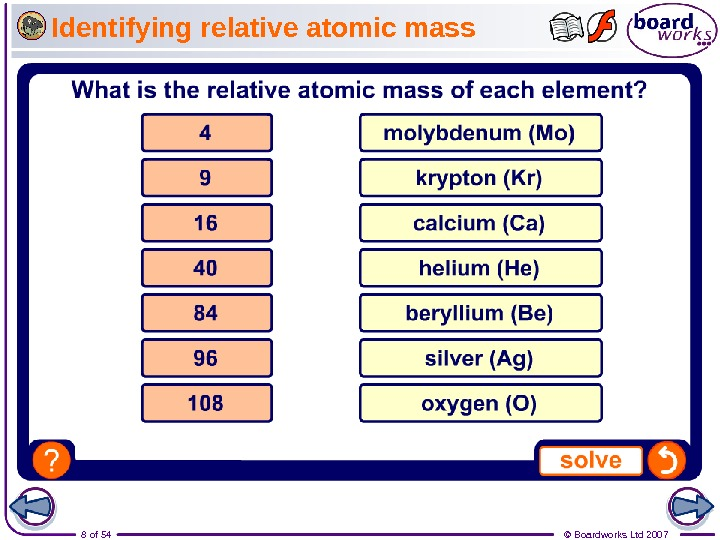8 of 54 © Boardworks Ltd 2007 Identifying relative atomic mass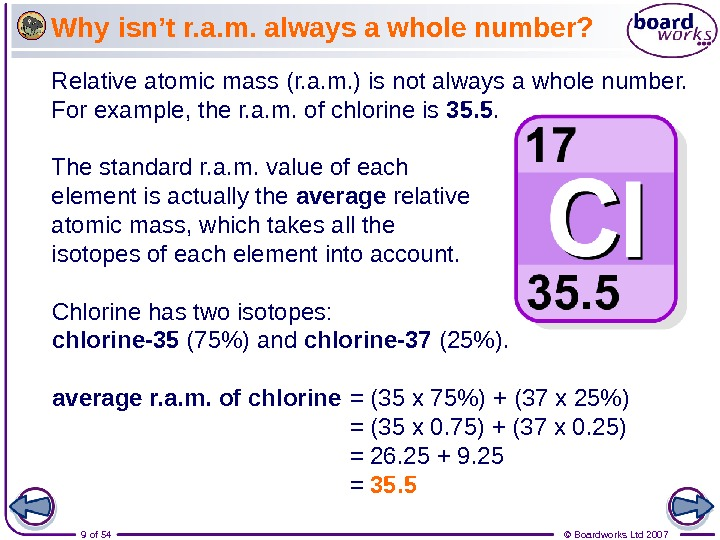9 of 54 © Boardworks Ltd 2007 Why isn’t r. a. m. always a whole number? Relative atomic mass (r. a. m. ) is not always a whole number. For example, the r. a. m. of chlorine is 35. 5. Chlorine has two isotopes: chlorine-35 (75%) and chlorine-37 (25%). The standard r. a. m. value of each element is actually the average relative atomic mass, which takes all the isotopes of each element into account. average r. a. m. of chlorine = (35 x 75%) + (37 x 25%) = (35 x 0. 75) + (37 x 0. 25) = 26. 25 + 9. 25 = 35.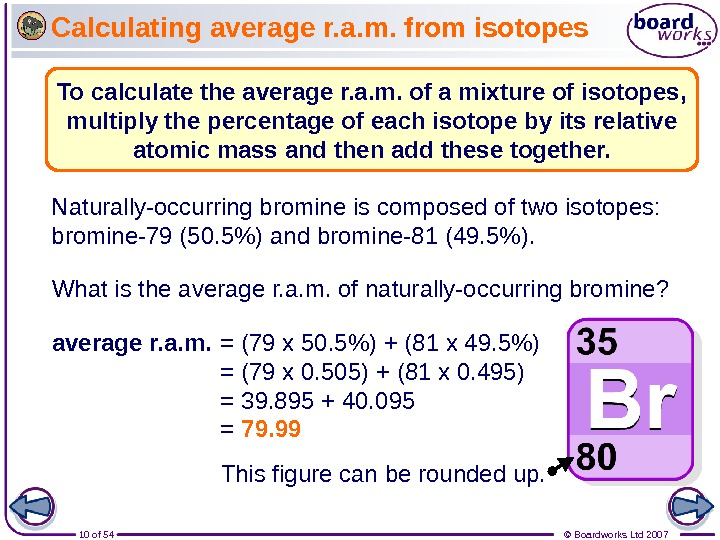10 of 54 © Boardworks Ltd 2007 Calculating average r. a. m. from isotopes What is the average r. a. m. of naturally-occurring bromine? Naturally-occurring bromine is composed of two isotopes: bromine-79 (50. 5%) and bromine-81 (49. 5%). average r. a. m. = (79 x 50. 5%) + (81 x 49. 5%) = (79 x 0. 505) + (81 x 0. 495) = 39. 895 + 40. 095 = 79. 99 This figure can be rounded up. To calculate the average r. a. m. of a mixture of isotopes, multiply the percentage of each isotope by its relative atomic mass and then add these together.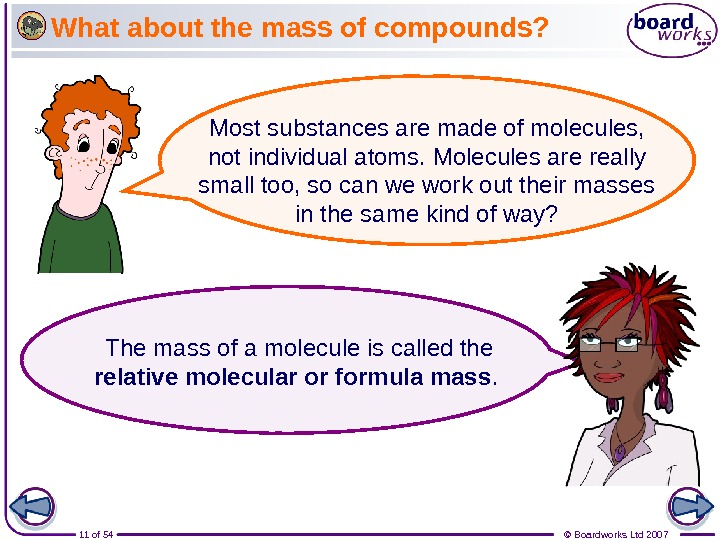11 of 54 © Boardworks Ltd 2007 What about the mass of compounds? Most substances are made of molecules, not individual atoms. Molecules are really small too, so can we work out their masses in the same kind of way? The mass of a molecule is called the relative molecular or formula mass.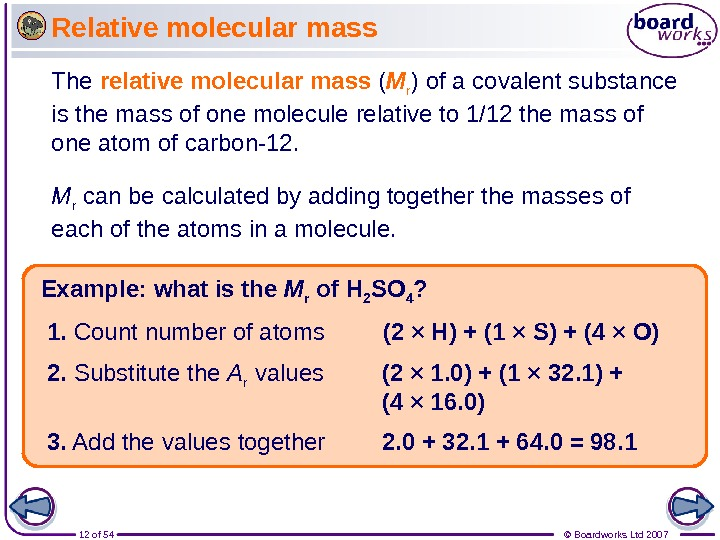12 of 54 © Boardworks Ltd 2007 Relative molecular mass Example: what is the M r of H 2 SO 4 ? (2 × H) + (1 × S) + (4 × O) 1. Count number of atoms (2 × 1. 0) + (1 × 32. 1) + (4 × 16. 0)2. Substitute the A r values 2. 0 + 32. 1 + 64. 0 = 98. 1 3. Add the values together The relative molecular mass ( M r ) of a covalent substance is the mass of one molecule relative to 1/12 the mass of one atom of carbon-12. M r can be calculated by adding together the masses of each of the atoms in a molecule.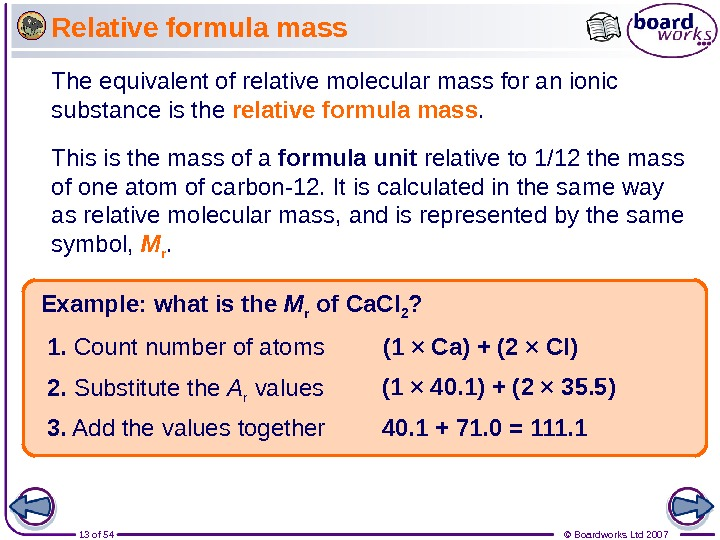13 of 54 © Boardworks Ltd 2007 Relative formula mass The equivalent of relative molecular mass for an ionic substance is the relative formula mass. This is the mass of a formula unit relative to 1/12 the mass of one atom of carbon-12. It is calculated in the same way as relative molecular mass, and is represented by the same symbol, M r. Example: what is the M r of Ca. Cl 2 ? (1 × Ca) + (2 × Cl) 1. Count number of atoms (1 × 40. 1) + (2 × 35. 5) 2. Substitute the A r values 40. 1 + 71. 0 = 111. 1 3. Add the values together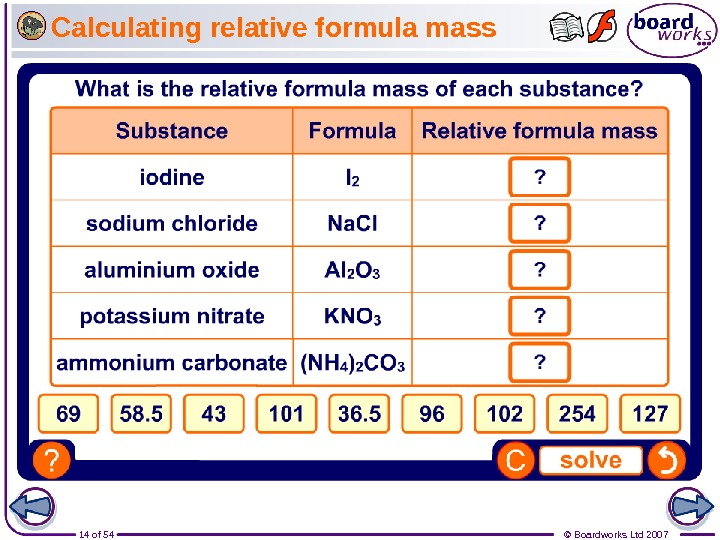14 of 54 © Boardworks Ltd 2007 Calculating relative formula mass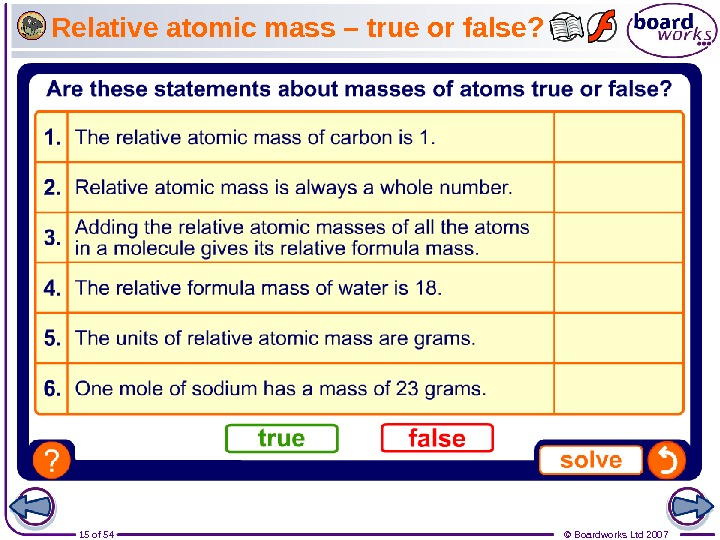15 of 54 © Boardworks Ltd 2007 Relative atomic mass – true or false?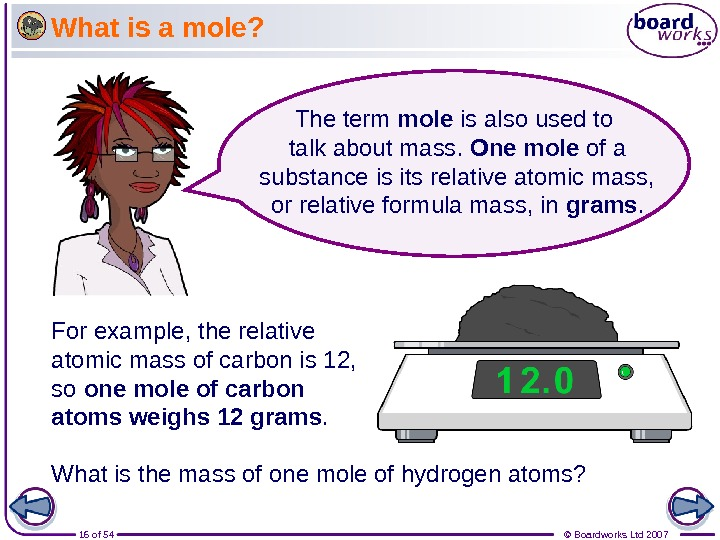16 of 54 © Boardworks Ltd 2007 What is a mole? For example, the relative atomic mass of carbon is 12, so one mole of carbon atoms weighs 12 grams. What is the mass of one mole of hydrogen atoms? The term mole is also used to talk about mass. One mole of a substance is its relative atomic mass, or relative formula mass, in grams.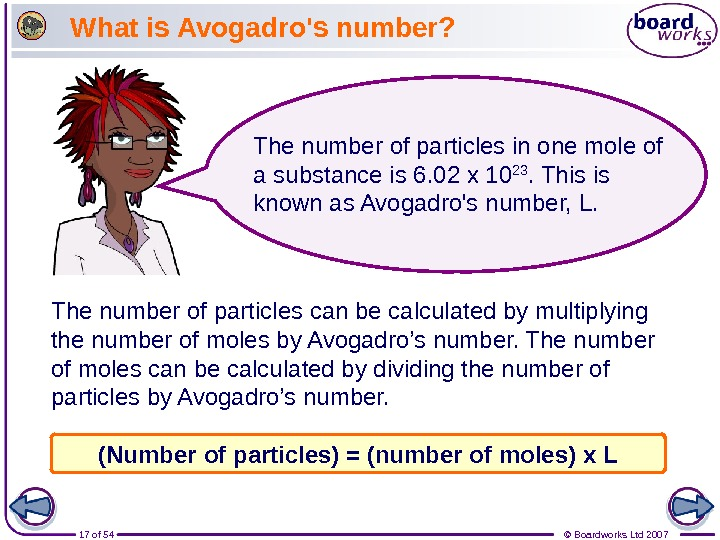17 of 54 © Boardworks Ltd 2007 What is Avogadro’s number? The number of particles can be calculated by multiplying the number of moles by Avogadro’s number. The number of moles can be calculated by dividing the number of particles by Avogadro’s number. The number of particles in one mole of a substance is 6. 02 x 10 23. This is known as Avogadro’s number, L. (Number of particles) = (number of moles) x L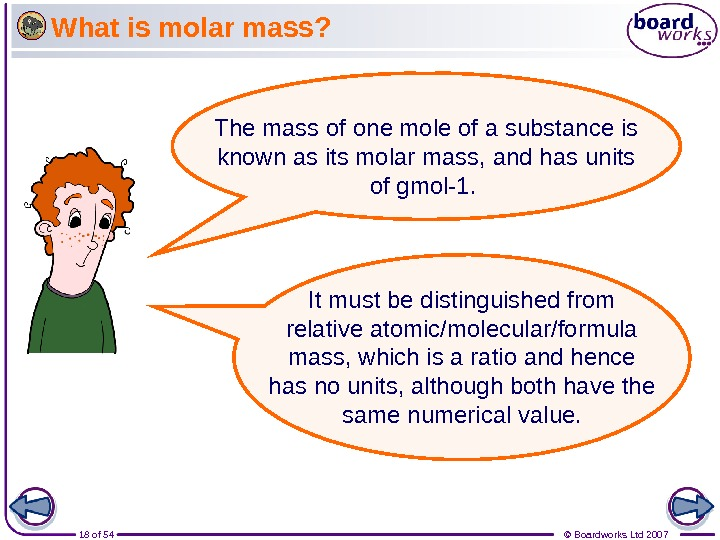18 of 54 © Boardworks Ltd 2007 What is molar mass? The mass of one mole of a substance is known as its molar mass, and has units of gmol-1. It must be distinguished from relative atomic/molecular/formula mass, which is a ratio and hence has no units, although both have the same numerical value.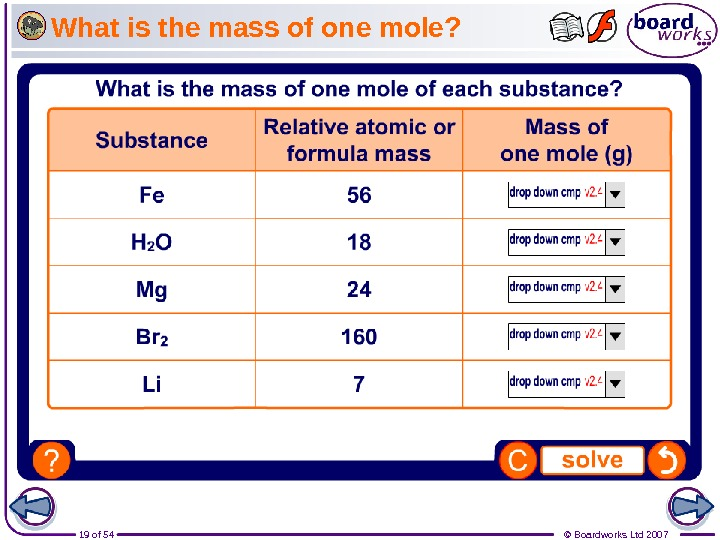19 of 54 © Boardworks Ltd 2007 What is the mass of one mole?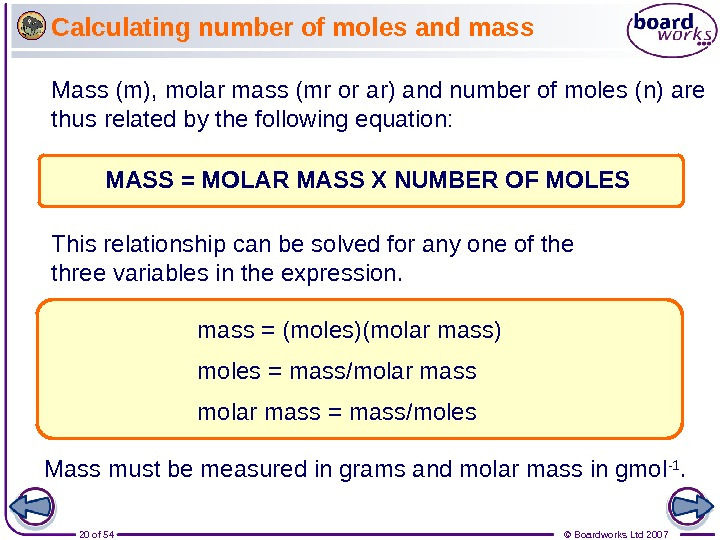20 of 54 © Boardworks Ltd 2007 Calculating number of moles and mass Mass (m), molar mass (mr or ar) and number of moles (n) are thus related by the following equation: MASS = MOLAR MASS X NUMBER OF MOLES mass = (moles)(molar mass) moles = mass/molar mass = mass/moles. This relationship can be solved for any one of the three variables in the expression. Mass must be measured in grams and molar mass in gmol -1.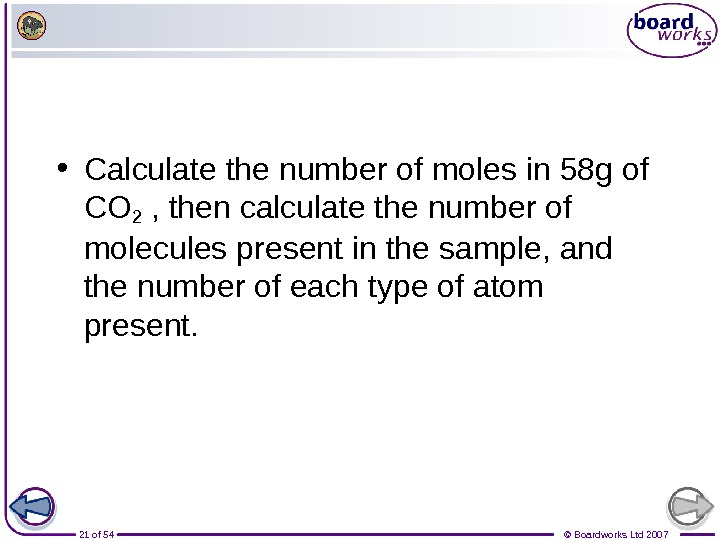21 of 54 © Boardworks Ltd 2007 • Calculate the number of moles in 58 g of CO 2 , then calculate the number of molecules present in the sample, and the number of each type of atom present.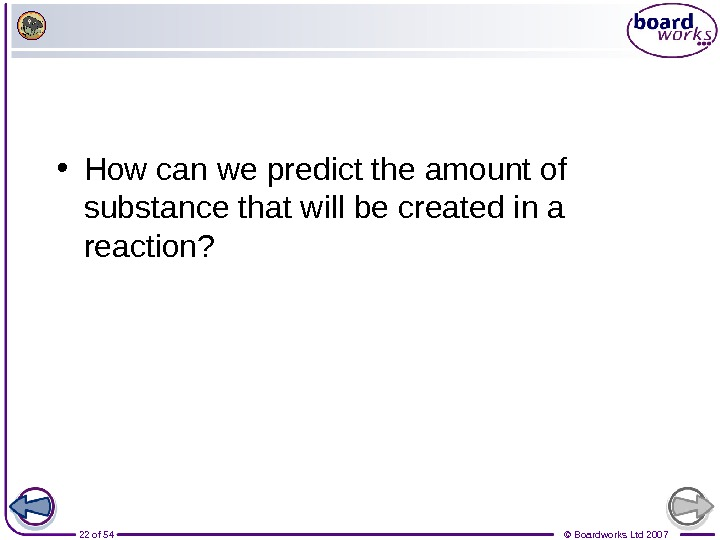22 of 54 © Boardworks Ltd 2007 • How can we predict the amount of substance that will be created in a reaction?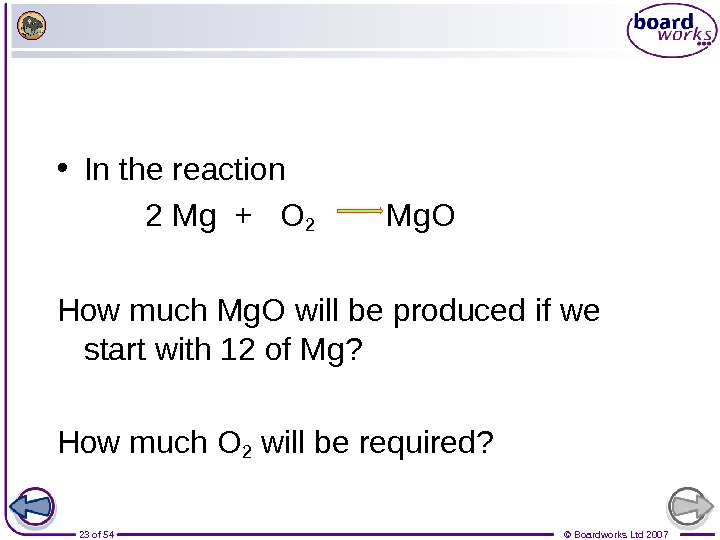23 of 54 © Boardworks Ltd 2007 • In the reaction 2 Mg + O 2 Mg. O How much Mg. O will be produced if we start with 12 of Mg? How much O 2 will be required?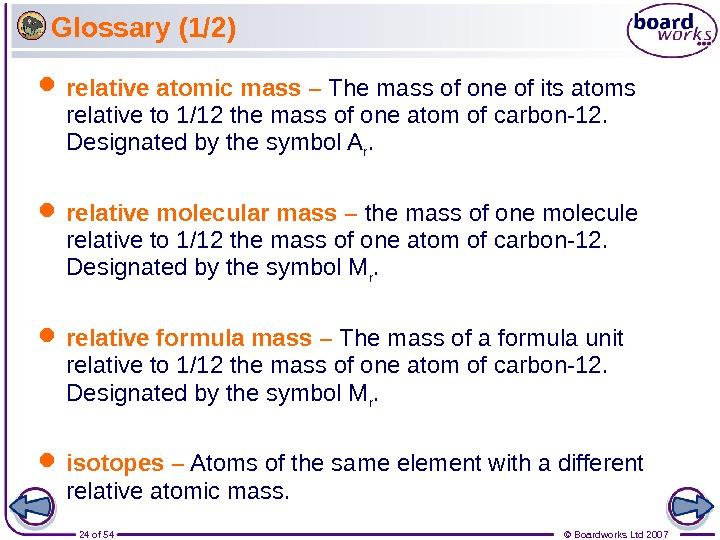24 of 54 © Boardworks Ltd 2007 Glossary (1/2) relative atomic mass – Th e mass of one of its atoms relative to 1/12 the mass of one atom of carbon-12. Designated by the symbol A r. relative molecular mass – the mass of one molecule relative to 1/12 the mass of one atom of carbon-12. Designated by the symbol M r. relative formula mass – The mass of a formula unit relative to 1/12 the mass of one atom of carbon-12. Designated by the symbol M r. isotopes – Atoms of the same element with a different relative atomic mass.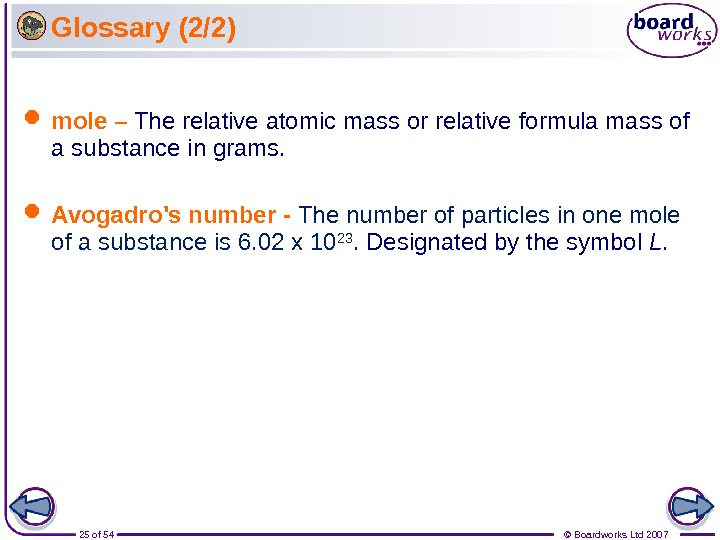25 of 54 © Boardworks Ltd 2007 Glossary (2/2) mole – The relative atomic mass or relative formula mass of a substance in grams. Avogadro’s number — The number of particles in one mole of a substance is 6. 02 x 10 23. Designated by the symbol L.